Chemistry 2e

# 3.1Formula Mass and the Mole Concept

Chemistry 2e3.1 Formula Mass and the Mole Concept

### Learning Objectives

By the end of this section, you will be able to:

• Calculate formula masses for covalent and ionic compounds
• Define the amount unit mole and the related quantity Avogadro’s number Explain the relation between mass, moles, and numbers of atoms or molecules, and perform calculations deriving these quantities from one another

Many argue that modern chemical science began when scientists started exploring the quantitative as well as the qualitative aspects of chemistry. For example, Dalton’s atomic theory was an attempt to explain the results of measurements that allowed him to calculate the relative masses of elements combined in various compounds. Understanding the relationship between the masses of atoms and the chemical formulas of compounds allows us to quantitatively describe the composition of substances.

### Formula Mass

An earlier chapter of this text described the development of the atomic mass unit, the concept of average atomic masses, and the use of chemical formulas to represent the elemental makeup of substances. These ideas can be extended to calculate the formula mass of a substance by summing the average atomic masses of all the atoms represented in the substance’s formula.

#### Formula Mass for Covalent Substances

For covalent substances, the formula represents the numbers and types of atoms composing a single molecule of the substance; therefore, the formula mass may be correctly referred to as a molecular mass. Consider chloroform (CHCl3), a covalent compound once used as a surgical anesthetic and now primarily used in the production of tetrafluoroethylene, the building block for the "anti-stick" polymer, Teflon. The molecular formula of chloroform indicates that a single molecule contains one carbon atom, one hydrogen atom, and three chlorine atoms. The average molecular mass of a chloroform molecule is therefore equal to the sum of the average atomic masses of these atoms. Figure 3.2 outlines the calculations used to derive the molecular mass of chloroform, which is 119.37 amu.

Figure 3.2 The average mass of a chloroform molecule, CHCl3, is 119.37 amu, which is the sum of the average atomic masses of each of its constituent atoms. The model shows the molecular structure of chloroform.

Likewise, the molecular mass of an aspirin molecule, C9H8O4, is the sum of the atomic masses of nine carbon atoms, eight hydrogen atoms, and four oxygen atoms, which amounts to 180.15 amu (Figure 3.3).

Figure 3.3 The average mass of an aspirin molecule is 180.15 amu. The model shows the molecular structure of aspirin, C9H8O4.

### Example 3.1

#### Computing Molecular Mass for a Covalent Compound

Ibuprofen, C13H18O2, is a covalent compound and the active ingredient in several popular nonprescription pain medications, such as Advil and Motrin. What is the molecular mass (amu) for this compound?

#### Solution

Molecules of this compound are composed of 13 carbon atoms, 18 hydrogen atoms, and 2 oxygen atoms. Following the approach described above, the average molecular mass for this compound is therefore: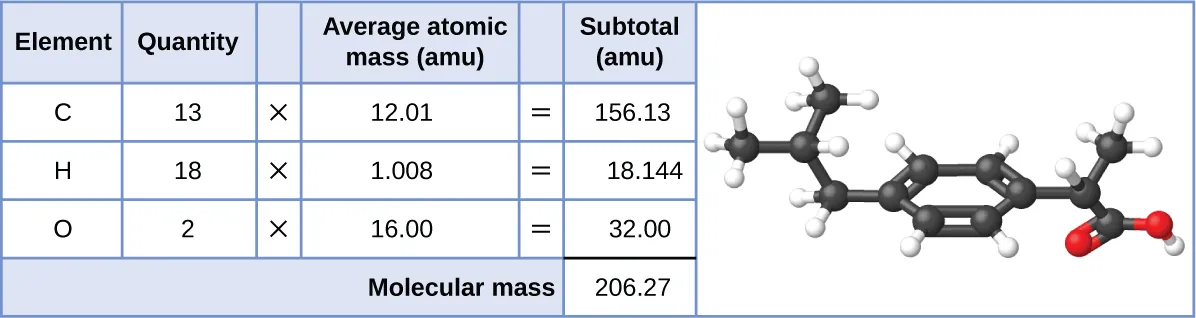Acetaminophen, C8H9NO2, is a covalent compound and the active ingredient in several popular nonprescription pain medications, such as Tylenol. What is the molecular mass (amu) for this compound?

151.16 amu

#### Formula Mass for Ionic Compounds

Ionic compounds are composed of discrete cations and anions combined in ratios to yield electrically neutral bulk matter. The formula mass for an ionic compound is calculated in the same way as the formula mass for covalent compounds: by summing the average atomic masses of all the atoms in the compound’s formula. Keep in mind, however, that the formula for an ionic compound does not represent the composition of a discrete molecule, so it may not correctly be referred to as the “molecular mass.”

As an example, consider sodium chloride, NaCl, the chemical name for common table salt. Sodium chloride is an ionic compound composed of sodium cations, Na+, and chloride anions, Cl, combined in a 1:1 ratio. The formula mass for this compound is computed as 58.44 amu (see Figure 3.4).

Figure 3.4 Table salt, NaCl, contains an array of sodium and chloride ions combined in a 1:1 ratio. Its formula mass is 58.44 amu.

Note that the average masses of neutral sodium and chlorine atoms were used in this computation, rather than the masses for sodium cations and chlorine anions. This approach is perfectly acceptable when computing the formula mass of an ionic compound. Even though a sodium cation has a slightly smaller mass than a sodium atom (since it is missing an electron), this difference will be offset by the fact that a chloride anion is slightly more massive than a chloride atom (due to the extra electron). Moreover, the mass of an electron is negligibly small with respect to the mass of a typical atom. Even when calculating the mass of an isolated ion, the missing or additional electrons can generally be ignored, since their contribution to the overall mass is negligible, reflected only in the nonsignificant digits that will be lost when the computed mass is properly rounded. The few exceptions to this guideline are very light ions derived from elements with precisely known atomic masses.

### Example 3.2

#### Computing Formula Mass for an Ionic Compound

Aluminum sulfate, Al2(SO4)3, is an ionic compound that is used in the manufacture of paper and in various water purification processes. What is the formula mass (amu) of this compound?

#### Solution

The formula for this compound indicates it contains Al3+ and SO42− ions combined in a 2:3 ratio. For purposes of computing a formula mass, it is helpful to rewrite the formula in the simpler format, Al2S3O12. Following the approach outlined above, the formula mass for this compound is calculated as follows: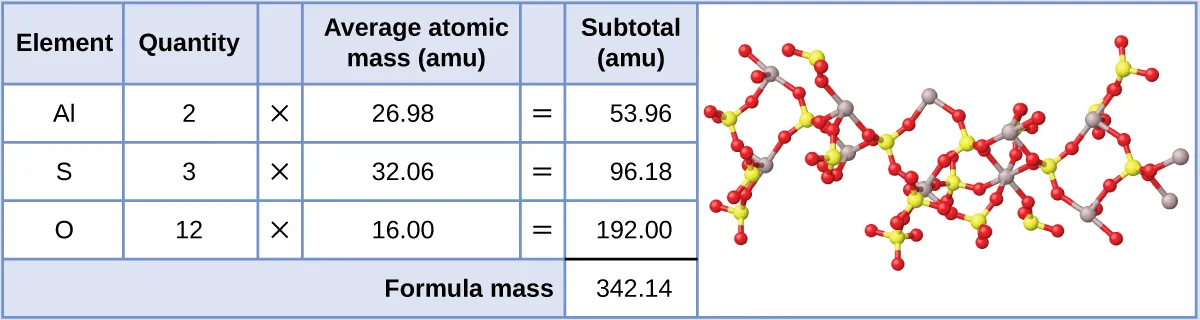Calcium phosphate, Ca3(PO4)2, is an ionic compound and a common anti-caking agent added to food products. What is the formula mass (amu) of calcium phosphate?

310.18 amu

### The Mole

The identity of a substance is defined not only by the types of atoms or ions it contains, but by the quantity of each type of atom or ion. For example, water, H2O, and hydrogen peroxide, H2O2, are alike in that their respective molecules are composed of hydrogen and oxygen atoms. However, because a hydrogen peroxide molecule contains two oxygen atoms, as opposed to the water molecule, which has only one, the two substances exhibit very different properties. Today, sophisticated instruments allow the direct measurement of these defining microscopic traits; however, the same traits were originally derived from the measurement of macroscopic properties (the masses and volumes of bulk quantities of matter) using relatively simple tools (balances and volumetric glassware). This experimental approach required the introduction of a new unit for amount of substances, the mole, which remains indispensable in modern chemical science.

The mole is an amount unit similar to familiar units like pair, dozen, gross, etc. It provides a specific measure of the number of atoms or molecules in a sample of matter. One Latin connotation for the word “mole” is “large mass” or “bulk,” which is consistent with its use as the name for this unit. The mole provides a link between an easily measured macroscopic property, bulk mass, and an extremely important fundamental property, number of atoms, molecules, and so forth. A mole of substance is that amount in which there are 6.02214076 $××$ 1023 discrete entities (atoms or molecules). This large number is a fundamental constant known as Avogadro’s number (NA) or the Avogadro constant in honor of Italian scientist Amedeo Avogadro. This constant is properly reported with an explicit unit of “per mole,” a conveniently rounded version being 6.022 $××$ 1023/mol.

Consistent with its definition as an amount unit, 1 mole of any element contains the same number of atoms as 1 mole of any other element. The masses of 1 mole of different elements, however, are different, since the masses of the individual atoms are drastically different. The molar mass of an element (or compound) is the mass in grams of 1 mole of that substance, a property expressed in units of grams per mole (g/mol) (see Figure 3.5).

Figure 3.5 Each sample contains 6.022 $××$ 1023 atoms —1.00 mol of atoms. From left to right (top row): 65.4 g zinc, 12.0 g carbon, 24.3 g magnesium, and 63.5 g copper. From left to right (bottom row): 32.1 g sulfur, 28.1 g silicon, 207 g lead, and 118.7 g tin. (credit: modification of work by Mark Ott)

The molar mass of any substance is numerically equivalent to its atomic or formula weight in amu. Per the amu definition, a single 12C atom weighs 12 amu (its atomic mass is 12 amu). A mole of 12C weighs 12 g (its molar mass is 12 g/mol). This relationship holds for all elements, since their atomic masses are measured relative to that of the amu-reference substance, 12C. Extending this principle, the molar mass of a compound in grams is likewise numerically equivalent to its formula mass in amu (Figure 3.6).

Figure 3.6 Each sample contains 6.02 $××$ 1023 molecules or formula units—1.00 mol of the compound or element. Clock-wise from the upper left: 130.2 g of C8H17OH (1-octanol, formula mass 130.2 amu), 454.4 g of HgI2 (mercury(II) iodide, formula mass 454.4 amu), 32.0 g of CH3OH (methanol, formula mass 32.0 amu) and 256.5 g of S8 (sulfur, formula mass 256.5 amu). (credit: Sahar Atwa)
Element Average Atomic Mass (amu) Molar Mass (g/mol) Atoms/Mole
C 12.01 12.01 6.022 $××$ 1023
H 1.008 1.008 6.022 $××$ 1023
O 16.00 16.00 6.022 $××$ 1023
Na 22.99 22.99 6.022 $××$ 1023
Cl 35.45 35.45 6.022 $××$ 1023

While atomic mass and molar mass are numerically equivalent, keep in mind that they are vastly different in terms of scale, as represented by the vast difference in the magnitudes of their respective units (amu versus g). To appreciate the enormity of the mole, consider a small drop of water weighing about 0.03 g (see Figure 3.7). Although this represents just a tiny fraction of 1 mole of water (~18 g), it contains more water molecules than can be clearly imagined. If the molecules were distributed equally among the roughly seven billion people on earth, each person would receive more than 100 billion molecules.

Figure 3.7 The number of molecules in a single droplet of water is roughly 100 billion times greater than the number of people on earth. (credit: “tanakawho”/Wikimedia commons)

The relationships between formula mass, the mole, and Avogadro’s number can be applied to compute various quantities that describe the composition of substances and compounds, as demonstrated in the next several example problems.

### Example 3.3

#### Deriving Moles from Grams for an Element

According to nutritional guidelines from the US Department of Agriculture, the estimated average requirement for dietary potassium is 4.7 g. What is the estimated average requirement of potassium in moles?

#### Solution

The mass of K is provided, and the corresponding amount of K in moles is requested. Referring to the periodic table, the atomic mass of K is 39.10 amu, and so its molar mass is 39.10 g/mol. The given mass of K (4.7 g) is a bit more than one-tenth the molar mass (39.10 g), so a reasonable “ballpark” estimate of the number of moles would be slightly greater than 0.1 mol.

The molar amount of a substance may be calculated by dividing its mass (g) by its molar mass (g/mol):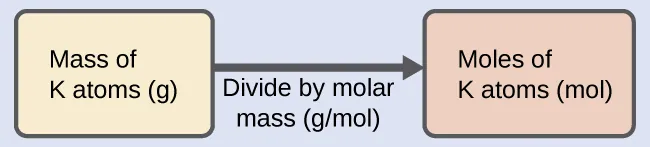The factor-label method supports this mathematical approach since the unit “g” cancels and the answer has units of “mol:”

$4.7gK(mol K39.10g K)=0.12mol K4.7gK(mol K39.10g K)=0.12mol K$

The calculated magnitude (0.12 mol K) is consistent with our ballpark expectation, since it is a bit greater than 0.1 mol.

Beryllium is a light metal used to fabricate transparent X-ray windows for medical imaging instruments. How many moles of Be are in a thin-foil window weighing 3.24 g?

0.360 mol

### Example 3.4

#### Deriving Grams from Moles for an Element

A liter of air contains 9.2 $××$ 10−4 mol argon. What is the mass of Ar in a liter of air?

#### Solution

The molar amount of Ar is provided and must be used to derive the corresponding mass in grams. Since the amount of Ar is less than 1 mole, the mass will be less than the mass of 1 mole of Ar, approximately 40 g. The molar amount in question is approximately one-one thousandth (~10−3) of a mole, and so the corresponding mass should be roughly one-one thousandth of the molar mass (~0.04 g):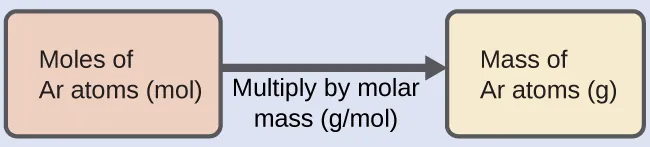In this case, logic dictates (and the factor-label method supports) multiplying the provided amount (mol) by the molar mass (g/mol):

$9.2×10−4molAr(39.95g ArmolAr)=0.037g Ar9.2×10−4molAr(39.95g ArmolAr)=0.037g Ar$

The result is in agreement with our expectations, around 0.04 g Ar.

What is the mass of 2.561 mol of gold?

504.4 g

### Example 3.5

#### Deriving Number of Atoms from Mass for an Element

Copper is commonly used to fabricate electrical wire (Figure 3.8). How many copper atoms are in 5.00 g of copper wire?
Figure 3.8 Copper wire is composed of many, many atoms of Cu. (credit: Emilian Robert Vicol)

#### Solution

The number of Cu atoms in the wire may be conveniently derived from its mass by a two-step computation: first calculating the molar amount of Cu, and then using Avogadro’s number (NA) to convert this molar amount to number of Cu atoms:Considering that the provided sample mass (5.00 g) is a little less than one-tenth the mass of 1 mole of Cu (~64 g), a reasonable estimate for the number of atoms in the sample would be on the order of one-tenth NA, or approximately 1022 Cu atoms. Carrying out the two-step computation yields:

$5.00gCu(molCu63.55g Cu)(6.022×1023Cu atomsmol Cu)=4.74×1022Cu atoms5.00gCu(molCu63.55g Cu)(6.022×1023Cu atomsmol Cu)=4.74×1022Cu atoms$

The factor-label method yields the desired cancellation of units, and the computed result is on the order of 1022 as expected.

A prospector panning for gold in a river collects 15.00 g of pure gold. How many Au atoms are in this quantity of gold?

4.586 $××$ 1022 Au atoms

### Example 3.6

#### Deriving Moles from Grams for a Compound

Our bodies synthesize protein from amino acids. One of these amino acids is glycine, which has the molecular formula C2H5O2N. How many moles of glycine molecules are contained in 28.35 g of glycine?

#### Solution

Derive the number of moles of a compound from its mass following the same procedure used for an element in Example 3.3: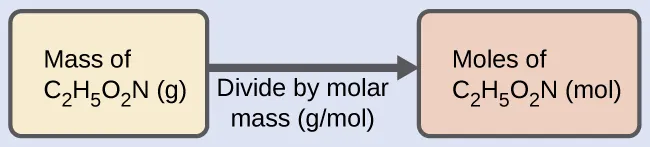The molar mass of glycine is required for this calculation, and it is computed in the same fashion as its molecular mass. One mole of glycine, C2H5O2N, contains 2 moles of carbon, 5 moles of hydrogen, 2 moles of oxygen, and 1 mole of nitrogen: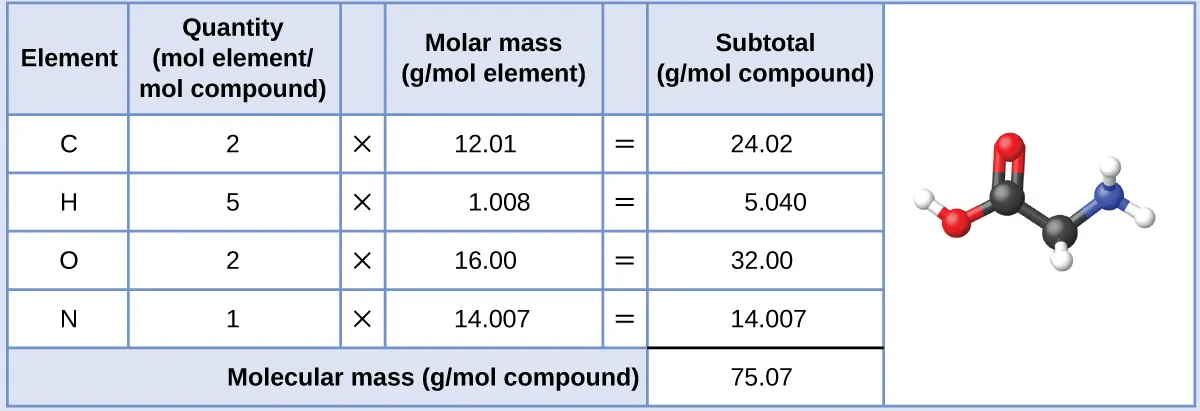The provided mass of glycine (~28 g) is a bit more than one-third the molar mass (~75 g/mol), so the computed result is expected to be a bit greater than one-third of a mole (~0.33 mol). Dividing the compound’s mass by its molar mass yields:

$28.35gglycine(mol glycine75.07g glycine)=0.378mol glycine28.35gglycine(mol glycine75.07g glycine)=0.378mol glycine$

This result is consistent with the rough estimate.

How many moles of sucrose, C12H22O11, are in a 25-g sample of sucrose?

0.073 mol

### Example 3.7

#### Deriving Grams from Moles for a Compound

Vitamin C is a covalent compound with the molecular formula C6H8O6. The recommended daily dietary allowance of vitamin C for children aged 4–8 years is 1.42 $××$ 10−4 mol. What is the mass of this allowance in grams?

#### Solution

As for elements, the mass of a compound can be derived from its molar amount as shown:The molar mass for this compound is computed to be 176.124 g/mol. The given number of moles is a very small fraction of a mole (~10−4 or one-ten thousandth); therefore, the corresponding mass is expected to be about one-ten thousandth of the molar mass (~0.02 g). Performing the calculation yields:

$1.42×10−4molvitamin C(176.124g vitamin Cmolvitamin C)=0.0250g vitamin C1.42×10−4molvitamin C(176.124g vitamin Cmolvitamin C)=0.0250g vitamin C$

This is consistent with the anticipated result.

What is the mass of 0.443 mol of hydrazine, N2H4?

14.2 g

### Example 3.8

#### Deriving the Number of Atoms and Molecules from the Mass of a Compound

A packet of an artificial sweetener contains 40.0 mg of saccharin (C7H5NO3S), which has the structural formula: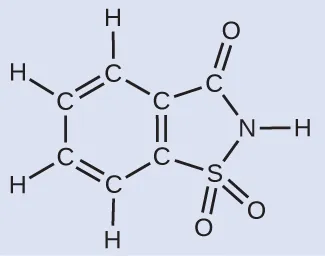Given that saccharin has a molar mass of 183.18 g/mol, how many saccharin molecules are in a 40.0-mg (0.0400-g) sample of saccharin? How many carbon atoms are in the same sample?

#### Solution

The number of molecules in a given mass of compound is computed by first deriving the number of moles, as demonstrated in Example 3.6, and then multiplying by Avogadro’s number: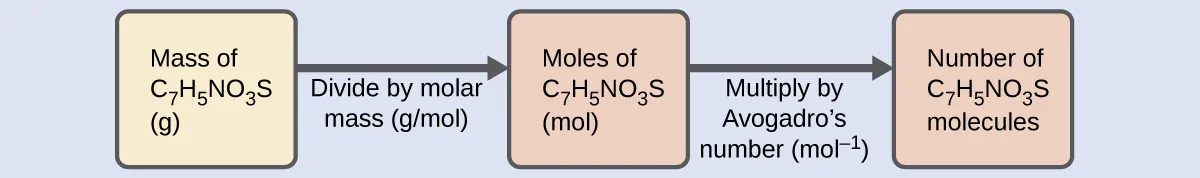Using the provided mass and molar mass for saccharin yields:

$0.0400gC7H5NO3S (molC7H5NO3S 183.18gC7H5NO3S )(6.022×1023C7H5NO3S molecules1molC7H5NO3S) =1.31×1020C7H5NO3Smolecules 0.0400gC7H5NO3S (molC7H5NO3S 183.18gC7H5NO3S )(6.022×1023C7H5NO3S molecules1molC7H5NO3S) =1.31×1020C7H5NO3Smolecules$

The compound’s formula shows that each molecule contains seven carbon atoms, and so the number of C atoms in the provided sample is:

$1.31×1020C7H5NO3S molecules(7C atoms1C7H5NO3S molecule)=9.17×1020C atoms1.31×1020C7H5NO3S molecules(7C atoms1C7H5NO3S molecule)=9.17×1020C atoms$

How many C4H10 molecules are contained in 9.213 g of this compound? How many hydrogen atoms?

9.545 $××$ 1022 molecules C4 H10; 9.545 $××$ 1023 atoms H

### How Sciences Interconnect

#### Counting Neurotransmitter Molecules in the Brain

The brain is the control center of the central nervous system (Figure 3.9). It sends and receives signals to and from muscles and other internal organs to monitor and control their functions; it processes stimuli detected by sensory organs to guide interactions with the external world; and it houses the complex physiological processes that give rise to our intellect and emotions. The broad field of neuroscience spans all aspects of the structure and function of the central nervous system, including research on the anatomy and physiology of the brain. Great progress has been made in brain research over the past few decades, and the BRAIN Initiative, a federal initiative announced in 2013, aims to accelerate and capitalize on these advances through the concerted efforts of various industrial, academic, and government agencies (more details available at www.whitehouse.gov/share/brain-initiative).

Figure 3.9 (a) A typical human brain weighs about 1.5 kg and occupies a volume of roughly 1.1 L. (b) Information is transmitted in brain tissue and throughout the central nervous system by specialized cells called neurons (micrograph shows cells at 1600× magnification).

Specialized cells called neurons transmit information between different parts of the central nervous system by way of electrical and chemical signals. Chemical signaling occurs at the interface between different neurons when one of the cells releases molecules (called neurotransmitters) that diffuse across the small gap between the cells (called the synapse) and bind to the surface of the other cell. These neurotransmitter molecules are stored in small intracellular structures called vesicles that fuse to the cell membrane and then break open to release their contents when the neuron is appropriately stimulated. This process is called exocytosis (see Figure 3.10). One neurotransmitter that has been very extensively studied is dopamine, C8H11NO2. Dopamine is involved in various neurological processes that impact a wide variety of human behaviors. Dysfunctions in the dopamine systems of the brain underlie serious neurological diseases such as Parkinson’s and schizophrenia.

Figure 3.10 (a) Chemical signals are transmitted from neurons to other cells by the release of neurotransmitter molecules into the small gaps (synapses) between the cells. (b) Dopamine, C8H11NO2, is a neurotransmitter involved in a number of neurological processes.

One important aspect of the complex processes related to dopamine signaling is the number of neurotransmitter molecules released during exocytosis. Since this number is a central factor in determining neurological response (and subsequent human thought and action), it is important to know how this number changes with certain controlled stimulations, such as the administration of drugs. It is also important to understand the mechanism responsible for any changes in the number of neurotransmitter molecules released—for example, some dysfunction in exocytosis, a change in the number of vesicles in the neuron, or a change in the number of neurotransmitter molecules in each vesicle.

Significant progress has been made recently in directly measuring the number of dopamine molecules stored in individual vesicles and the amount actually released when the vesicle undergoes exocytosis. Using miniaturized probes that can selectively detect dopamine molecules in very small amounts, scientists have determined that the vesicles of a certain type of mouse brain neuron contain an average of 30,000 dopamine molecules per vesicle (about $5×10−205×10−20$ mol or 50 zmol). Analysis of these neurons from mice subjected to various drug therapies shows significant changes in the average number of dopamine molecules contained in individual vesicles, increasing or decreasing by up to three-fold, depending on the specific drug used. These studies also indicate that not all of the dopamine in a given vesicle is released during exocytosis, suggesting that it may be possible to regulate the fraction released using pharmaceutical therapies.1

### Footnotes

• 1Omiatek, Donna M., Amanda J. Bressler, Ann-Sofie Cans, Anne M. Andrews, Michael L. Heien, and Andrew G. Ewing. “The Real Catecholamine Content of Secretory Vesicles in the CNS Revealed by Electrochemical Cytometry.” Scientific Report 3 (2013): 1447, accessed January 14, 2015, doi:10.1038/srep01447.
Order a print copy

As an Amazon Associate we earn from qualifying purchases.